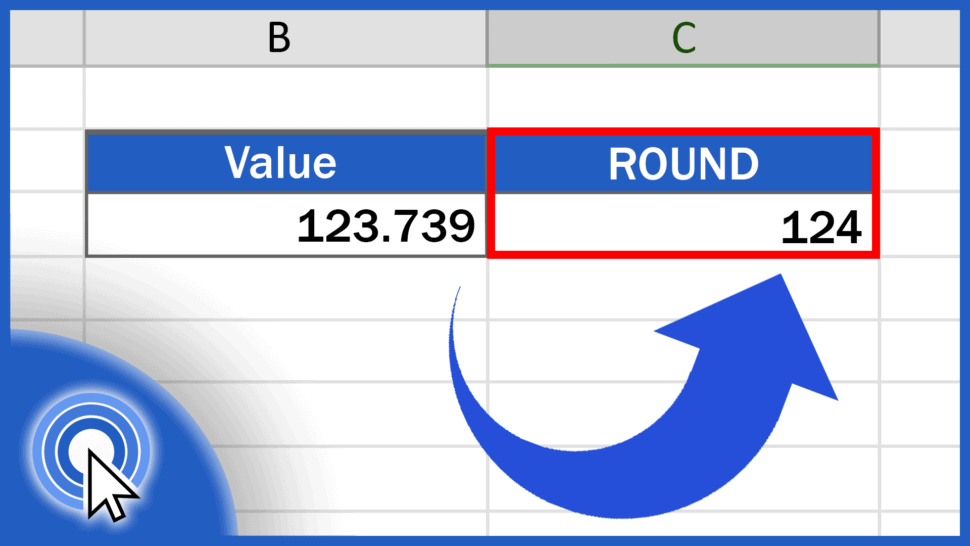# How to ROUND Numbers in ExcelIn this tutorial, we’re gonna go through a couple of steps on how to round numbers in Excel.

Thanks to the ROUND function, Excel will round values to a given number of decimal places based on the general mathematical rule. This means that if the next decimal place is 4 or less, it will round the value down, but if it’s 5 or more, it will round it up.

Let’s see how to use this function effectively in more detail.

See the video tutorial and transcription below:

Contents

As we already know, Excel offers multiple functions when it comes to calculations of any kind. The same goes for rounding numbers. We can round up, round down, or simply round numbers according to the general mathematical rule. Each function will help you round numbers in a different way and each is going to be presented in a separate tutorial. You’ll find the links to these videos in the description below.

In this tutorial, we’re gonna have a closer look at the function ROUND.

## How to ROUND to one decimal place in Excel

Let’s say we want to round the value in the column B mathematically, to various numbers of decimal places or to make it a whole number.

Click into the cell C3, enter the equal sign and type in the function ‘ROUND’. Then carry on with an opening bracket and here we’ll need to include two things.

The first one will be the cell which contains the number we want to round, so click on B3, then enter a comma and you can type in the second value – the number which identifies how many decimal places there should be in the result.

So, if you want to round the number to one decimal place, type ‘1’, close the parentheses, and hit ‘Enter’.

In C3, Excel will show the result – the number from B3 rounded to one decimal place according to the standard mathematical rule for rounding, which in this case will be the number 123.8.

The digit that ‘decided’ which way the number should be rounded was 5 – that’s why it was rounded up.

If the ‘deciding number’ had been 3, Excel would’ve rounded the value down to 123.7. That’s because the number 3 fits within the range of 0 to 4 and in that case, we would’ve done a round-down.

But let’s move on, now.

## How to ROUND to two decimal places in Excel

If you want to round a number to two decimal places, simply use 2 in the function and

the resulting number will be rounded mathematically to two decimal places.

By using positive numbers like 1, 2, 3, and so on, you can round up or down to any number of decimal places.

We’ll carry on now and discuss some more scenarios.

## How to round the value so that you would make it a whole number

To round the value so that you would make it a whole number, enter a zero in the function, and Excel will do just what you want – it will round the original value mathematically making it a whole number

like this:

And we’re not finished yet!

## How to round a number to tens or hundreds

To round a number to tens or hundreds, which means moving to the left from the decimal point, you need to use negative numbers in the formula, so make sure you include the minus sign with the number in parentheses.

Here are a couple of examples.

To round the number to the nearest ten, enter ‘-1’ into the formula

and you’ll see the result 120, because the ‘deciding number’ here is 3 – a number between 0 and 4. So the value has been rounded down.

Still not all for today!

If you want to round the number to the nearest hundred, type in ‘-2’ and Excel will calculate it for you immediately.

And that’s how it works! You can use this function to round any number just as you need, based on the mathematical rule for rounding.

If you’d like to know more about similar functions, like the function Round Up or Round Down, you can learn how to use them effectively by watching the rest of the video tutorials on rounding numbers by EasyClick Academy. Check out the links below!

If you found this tutorial helpful, give us a like and watch other video tutorials by EasyClick Academy. Learn how to use Excel in a quick and easy way!

Is this your first time on EasyClick? We’ll be more than happy to welcome you in our online community. Hit that Subscribe button and join the EasyClickers!

Thanks for watching and I’ll see you in the next tutorial!